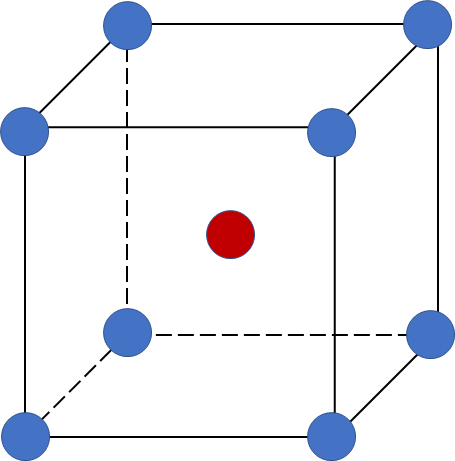# Problem: Chromium crystallizes with a body-centered cubic unit cell. The radius of a chromium atom is 125 pm. Calculate the density of solid crystalline chromium in grams per cubic centimeter.

###### FREE Expert Solution

A body-centered cubic (BCC) unit cell is composed of a cube with one atom at each of its corners and one atom at the center of the cubeRecall that the edge length (a) of a BCC unit cell can be calculated using the equation:

$\overline{){\mathbf{a}}{\mathbf{=}}\frac{\mathbf{4}\mathbf{r}}{\sqrt{\mathbf{3}}}}$

Step 1: Calculate the volume of 1 unit cell using density

• molar mass Cr = 51.99 g/mol
• 1 mole = 6.022x1023 entities (Avogadro' number)
entities = atoms, ions, molecules, formula units

Recall the # of atoms present per 1 BCC unit cell: corner atoms contribute 1/8 and the center atom contribute 1

# of atoms = 2 per 1 unit cell

Solving volume of 1 unit cell:

volume of unit cell = 2.406 x 10-23 cm3

84% (153 ratings)###### Problem Details

Chromium crystallizes with a body-centered cubic unit cell. The radius of a chromium atom is 125 pm. Calculate the density of solid crystalline chromium in grams per cubic centimeter.